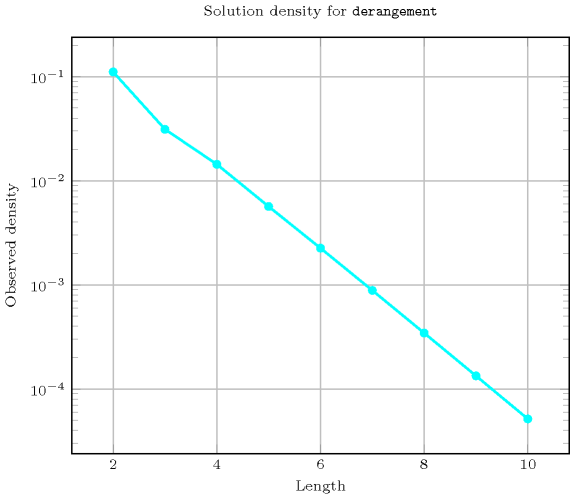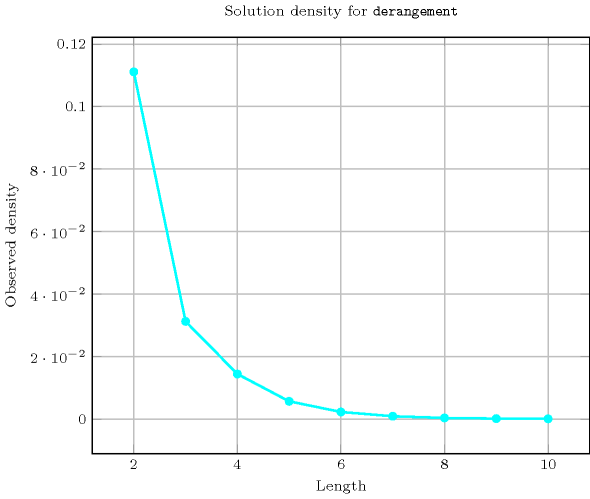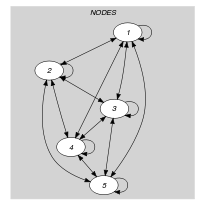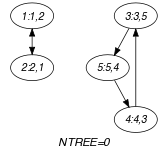## 5.114. derangement

Origin

Derived from $\mathrm{𝚌𝚢𝚌𝚕𝚎}$.

Constraint

$\mathrm{𝚍𝚎𝚛𝚊𝚗𝚐𝚎𝚖𝚎𝚗𝚝}\left(\mathrm{𝙽𝙾𝙳𝙴𝚂}\right)$

Argument
 $\mathrm{𝙽𝙾𝙳𝙴𝚂}$ $\mathrm{𝚌𝚘𝚕𝚕𝚎𝚌𝚝𝚒𝚘𝚗}\left(\mathrm{𝚒𝚗𝚍𝚎𝚡}-\mathrm{𝚒𝚗𝚝},\mathrm{𝚜𝚞𝚌𝚌}-\mathrm{𝚍𝚟𝚊𝚛}\right)$
Restrictions
 $|\mathrm{𝙽𝙾𝙳𝙴𝚂}|>1$ $\mathrm{𝚛𝚎𝚚𝚞𝚒𝚛𝚎𝚍}$$\left(\mathrm{𝙽𝙾𝙳𝙴𝚂},\left[\mathrm{𝚒𝚗𝚍𝚎𝚡},\mathrm{𝚜𝚞𝚌𝚌}\right]\right)$ $\mathrm{𝙽𝙾𝙳𝙴𝚂}.\mathrm{𝚒𝚗𝚍𝚎𝚡}\ge 1$ $\mathrm{𝙽𝙾𝙳𝙴𝚂}.\mathrm{𝚒𝚗𝚍𝚎𝚡}\le |\mathrm{𝙽𝙾𝙳𝙴𝚂}|$ $\mathrm{𝚍𝚒𝚜𝚝𝚒𝚗𝚌𝚝}$$\left(\mathrm{𝙽𝙾𝙳𝙴𝚂},\mathrm{𝚒𝚗𝚍𝚎𝚡}\right)$ $\mathrm{𝙽𝙾𝙳𝙴𝚂}.\mathrm{𝚜𝚞𝚌𝚌}\ge 1$ $\mathrm{𝙽𝙾𝙳𝙴𝚂}.\mathrm{𝚜𝚞𝚌𝚌}\le |\mathrm{𝙽𝙾𝙳𝙴𝚂}|$
Purpose

Enforce to have a permutation with no cycle of length one. The permutation is depicted by the $\mathrm{𝚜𝚞𝚌𝚌}$ attribute of the $\mathrm{𝙽𝙾𝙳𝙴𝚂}$ collection.

Example
$\left(\begin{array}{c}〈\begin{array}{cc}\mathrm{𝚒𝚗𝚍𝚎𝚡}-1\hfill & \mathrm{𝚜𝚞𝚌𝚌}-2,\hfill \\ \mathrm{𝚒𝚗𝚍𝚎𝚡}-2\hfill & \mathrm{𝚜𝚞𝚌𝚌}-1,\hfill \\ \mathrm{𝚒𝚗𝚍𝚎𝚡}-3\hfill & \mathrm{𝚜𝚞𝚌𝚌}-5,\hfill \\ \mathrm{𝚒𝚗𝚍𝚎𝚡}-4\hfill & \mathrm{𝚜𝚞𝚌𝚌}-3,\hfill \\ \mathrm{𝚒𝚗𝚍𝚎𝚡}-5\hfill & \mathrm{𝚜𝚞𝚌𝚌}-4\hfill \end{array}〉\hfill \end{array}\right)$

In the permutation of the example we have the following 2 cycles: $1\to 2\to 1$ and $3\to 5\to 4\to 3$. Since these cycles have both a length strictly greater than one the corresponding $\mathrm{𝚍𝚎𝚛𝚊𝚗𝚐𝚎𝚖𝚎𝚗𝚝}$ constraint holds.

Typical
$|\mathrm{𝙽𝙾𝙳𝙴𝚂}|>2$
Symmetries
• Items of $\mathrm{𝙽𝙾𝙳𝙴𝚂}$ are permutable.

• Attributes of $\mathrm{𝙽𝙾𝙳𝙴𝚂}$ are permutable w.r.t. permutation $\left(\mathrm{𝚒𝚗𝚍𝚎𝚡},\mathrm{𝚜𝚞𝚌𝚌}\right)$ (permutation applied to all items).

Remark

A special case of the $\mathrm{𝚌𝚢𝚌𝚕𝚎}$ [BeldiceanuContejean94] constraint.

Counting
 Length ($n$) 2 3 4 5 6 7 8 9 10 Solutions 1 2 9 44 265 1854 14833 133496 1334961

Number of solutions for $\mathrm{𝚍𝚎𝚛𝚊𝚗𝚐𝚎𝚖𝚎𝚗𝚝}$: domains $0..n$Keywords
Cond. implications

$\mathrm{𝚍𝚎𝚛𝚊𝚗𝚐𝚎𝚖𝚎𝚗𝚝}\left(\mathrm{𝙽𝙾𝙳𝙴𝚂}\right)$

implies $\mathrm{𝚙𝚎𝚛𝚖𝚞𝚝𝚊𝚝𝚒𝚘𝚗}$$\left(\mathrm{𝚅𝙰𝚁𝙸𝙰𝙱𝙻𝙴𝚂}:\mathrm{𝙽𝙾𝙳𝙴𝚂}\right)$.

Arc input(s)

$\mathrm{𝙽𝙾𝙳𝙴𝚂}$

Arc generator
$\mathrm{𝐶𝐿𝐼𝑄𝑈𝐸}$$↦\mathrm{𝚌𝚘𝚕𝚕𝚎𝚌𝚝𝚒𝚘𝚗}\left(\mathrm{𝚗𝚘𝚍𝚎𝚜}\mathtt{1},\mathrm{𝚗𝚘𝚍𝚎𝚜}\mathtt{2}\right)$

Arc arity
Arc constraint(s)
 $•\mathrm{𝚗𝚘𝚍𝚎𝚜}\mathtt{1}.\mathrm{𝚜𝚞𝚌𝚌}=\mathrm{𝚗𝚘𝚍𝚎𝚜}\mathtt{2}.\mathrm{𝚒𝚗𝚍𝚎𝚡}$ $•\mathrm{𝚗𝚘𝚍𝚎𝚜}\mathtt{1}.\mathrm{𝚜𝚞𝚌𝚌}\ne \mathrm{𝚗𝚘𝚍𝚎𝚜}\mathtt{1}.\mathrm{𝚒𝚗𝚍𝚎𝚡}$
Graph property(ies)
$\mathrm{𝐍𝐓𝐑𝐄𝐄}$$=0$

Graph class
$\mathrm{𝙾𝙽𝙴}_\mathrm{𝚂𝚄𝙲𝙲}$

Graph model

Parts (A) and (B) of Figure 5.114.1 respectively show the initial and final graph associated with the Example slot. The $\mathrm{𝚍𝚎𝚛𝚊𝚗𝚐𝚎𝚖𝚎𝚗𝚝}$ constraint holds since the final graph does not contain any vertex that does not belong to a circuit (i.e., $\mathrm{𝐍𝐓𝐑𝐄𝐄}$ $=$ 0).

##### Figure 5.114.1. Initial and final graph of the $\mathrm{𝚍𝚎𝚛𝚊𝚗𝚐𝚎𝚖𝚎𝚗𝚝}$ constraint(a) (b)

In order to express the binary constraint that links two vertices of the $\mathrm{𝙽𝙾𝙳𝙴𝚂}$ collection one has to make explicit the index value of the vertices. This is why the $\mathrm{𝚍𝚎𝚛𝚊𝚗𝚐𝚎𝚖𝚎𝚗𝚝}$ constraint considers objects that have two attributes:

• One fixed attribute $\mathrm{𝚒𝚗𝚍𝚎𝚡}$ that is the identifier of the vertex,

• One variable attribute $\mathrm{𝚜𝚞𝚌𝚌}$ that is the successor of the vertex.

Forbidding cycles of length one is achieved by the second condition of the arc constraint.

Signature

Since 0 is the smallest possible value of $\mathrm{𝐍𝐓𝐑𝐄𝐄}$ we can rewrite the graph property $\mathrm{𝐍𝐓𝐑𝐄𝐄}$ $=$ 0 to $\mathrm{𝐍𝐓𝐑𝐄𝐄}$ $\le$ 0. This leads to simplify $\underline{\overline{\mathrm{𝐍𝐓𝐑𝐄𝐄}}}$ to $\underline{\mathrm{𝐍𝐓𝐑𝐄𝐄}}$.